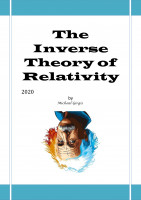# The Properties of Parallel Energy Dimensions

After we presented in the previous research, the concept of parallel energy dimensions, we present in this research the properties of parallel energy dimensions, and through the properties, we find that the relativistic variables in the special theory of relativity such as mass, time, and work done, these variables can occur in nature in an inverse way as well More
Price: Free! USD
Tags:
About the Series: The Inverse Theory of RelativityThe series includes 5 papers to explain a new theory called the inverse theory of relativity, The new theory maintains the principles, postulates, and results on which The special and general theories of relativity depend, The new theory is based on an observation process that is not followed by previous theories, Through the new state of observation, We try to reveal another aspect of relativity in which the results are opposite to the results known in previous theories
- the inverse relativity introduces new concepts such as The Energy Dimensions, Parallel Mass, Parallel Time, Super Time, Negative Work Done , Spacetime split into positive and negative
- The theory is simple and not complicated mathematically, linear equations and tensor rank one (i.e., a vector) , and there is more than one proof of the same results.
- Read the series in the following order
- Special Relativity Error .1, Energy and Time Paradox
- Modified Lorentz Transformations
- Parallel Energy Dimensions
- The Properties of Parallel Energy Dimensions
- Test The Theory, Dimensional Photons Accelerator

## Reviews

This book has not yet been reviewed.